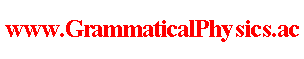since 2006 Help　Sitemap < Forum > < Problems > < Quantum History Theory > < New Quantum Grammar > When φ is a time dependent wave function in the old quantum mechanics, a quantum history corresponding to it is represented by a functional Φ defined schematically as follows. Φ[χ] = Πtφ(χ(t), t). In the old quantum mechanics, a time dependent wave function gives a probability distribution of the first measurement. Once the first measurement is done, the wave function is no longer the same. The above functional for a quantum history can not give a correct predictions about the relation between the results of measurements performed at different times. My assumption is that the entanglement involved in a quantum history yields the relation between the results of measurements performed at different times. The quantum history expressed by the above functional is not entangled, and so it can not yield such an relation. There may be two kinds of entanglement. The quantum history expressed by the above functional can not be a solution of the Uda equation. So, every solution of the Uda equation is entangled. This is the first kind of entanglement. Any sum of solutions of the Uda equation is a solution of the Uda equation. So, a solution of the Uda equation is generally entangled. This is the second kind of entanglement. I think that what yields the relation between measurement is the second kind of entanglement. Because the wave function is no longer the same after the first measurement. Author Yuichi Uda, Write start at 2016/04/17/19:42JST, Last edit at 2016/04/18/17:11JST